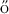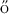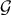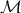Meeting in honor of András Sebő

April 24-25, 2014, Grenoble, France

Schedule

Thursday 24th April

 9:30-10:00 Registration Morning session 10:00-10:15 Zoltán Szigeti Introduction 10:15-10:45 András Frank On parity and submodularity Parity and submodularity are two major aspects of combinatorial optimization. In this talk, I discuss two unrelated results: an old lemma from parity and a recent application of submodularity. A parity-related fundamental result concerning minimal T-joins is Seb’s lemma on conservative ±1-weightings. Mathematical Reviews writes about this result as follows. One of the lasting contributions of this paper may be its identification of a disarmingly simple property of bipartite graphs as a source of the theory. I recall the birth of Seb’s lemma and briefly overview how I teach it in these days in a regular course. In the second part, as a recent application of submodularity, it will be shown that the problem of finding k disjoint spanning arborescences of a given root with specified number of root-edges can be treated with the help of a min-max theorem on covering crossing supermodular bi-set functions by digraphs. 10:45-11:15 Beth Novick Location Functions on Finite Metric SpacesA median of a sequence π = x1,x2,…,xk of elements of a finite metric space (X,d) is an element x minimizing ∑ i=1kd(x,x i). The median function M is defined on the set of all finite sequences on X by M(π) = {x : x is a median of π}. A median graph is a graph for which every profile of length 3 has a unique median. Median graphs have been well studied, possess a beautiful structure and arise in many arenas, including ternary algebra, ordered sets and discrete distributed lattices. Trees and hypercubes are key examples of median graphs. Recently Mulder and Novick, 2013, settled a question originally posed in 1994, by McMorris, Mulder and Roberts, by characterizing the median function on median graphs. Only three surprisingly simple axioms were used: Anonymity (A), Betweenness (B) and Consistency (C). Indeed, these three axioms hold for median functions on every metric space. Natural questions arise: Are (A), (B) and (C) independent? What is the relationship between this ‘ABC Theorem’ and an earlier less intuitively appealing characterization, due to McMorris, Mulder and Powers. For which metric spaces do (A), (B) and (C) characterize the median function? For a class of graphs,, what functions satisfy (A), (B) and (C) – what other axioms are needed? We settle the first two questions and offer preliminary remarks and conjectures for the latter two. Coffee Break 11:45-12:15 Michael Tarsi The hunting of the snark "Snarks" refers to Non-three-edge-colorable cubic graphs (some say, also 4-cyclically edge connected), so named by Martin Gardner on 1976, to emphasize the rarity and evasive nature of these mysterious creatures. Although indeed rare in some sense (almost all cubic graphs are Hamiltonian), Snarks are not really hard to "hunt", not anymore. Many infinite families of Snarks are now known; thousands individual Snarks were explicitly listed and studied and their number is constantly growing. For one, being a Snark is, in general, NP-Hard to detect, which does suggest certain structural richness and elusiveness. Back on 1998 we (Goddyn, Tarsi and Zhang) introduced the notion of Fractional (a.k.a. Circular) Nowhere-Zero-Flows. Apparently, the "Circular-flow number" of a Snark G, Cf(G), provides a reasonable quantitative measure of its Snarkness: For a 3-edge-colorable cubic graph G, Cf(G)=4, and recently (2011), Lukot'ka and Skoviera constructed an infinite set of Snarks G with Cf(G)= q, for every rational 4

Friday 25th April

 Morning session 9:00-9:30 Jens Vygen Approximation algorithms for the traveling salesman: recent advances and future directions35 years after Christofides' algorithm, this topic has received again a lot of interest recently. Since 2010, different approximation algorithms have been proposed for the asymmetric TSP, the graph-TSP, and the s-t path TSP. However, we still do not know the integrality ratios and even less the approximability, and we have still not beaten Christofides. What could be the next steps? 9:30-10:00 Nicolas Trotignon Isolating highly connected induced subgraphs When G is a graph, we denote by δ(G) the minimum degree of G. If H is an induced subgraph of G, then ∂(H) is the set of vertices of H that have neighbors outside of H. We prove the following theorem. Let k be a positive integer, and G be a graph. If δ(G) > 2k2 - 1, then G contains a (k + 1)-connected induced subgraph H such that ∂(H) ⊊ V (H) and |∂(H)|≤ 2k2 - 1. This may be seen as a generalization of a classical result of Mader. We will try to convince the audience that this theorem is meaningful in structural graph theory. We will discuss several variants, and for each of them how the bounds can be improved. We will see how to use our theorem to prove that gluing graphs with bounded chromatic number along a fixed number of vertices keeps the chromatic number bounded. This is joint work with Irena Penev and Stéphan Thomassé. 10:00-10:30 Gábor Bacsó Old and young – perfect graphs and their cliques Though the Perfect Graph Conjecture has become a theorem, many amazing open problems remained in the area. We discuss only two of them in the talk. A(n arbitrary) graph is uniquely colourable if there is only one partition of the vertex set yielding a minimum (proper) vertex colouring. Denoting the maximum size of a clique in G by ω(G) = ω, a clique pair is a subgraph having ω + 1 vertices and exactly one non-edge. The weakest conjecture in the subject is the Clique Pair Conjecture: Every non-clique uniquely colourable perfect graph has a clique pair. Dropping the condition of perfectness, the statement is not true even for ω(G) = 3. The other group of problem is more familiar and popular nowadays. The following concepts are well-known but we define them here. Let(G) be the hypergraph on V (G), constructed from the vertex sets, spanning a maximal clique of (an arbitrary) G. The clique chromatic number χC(G) is the (traditional) chromatic number of(G). Anonymous Conjecture: There exists a constant c such that χC(G) ≤ c for every perfect graph G. For general graphs this is false, similarly as above. In the Anonymous Conjecture, even c = 3 is possible. I hope I will have enough time to tell also about the clique chromatic number of line graphs. You conjecture that we were working together with András on the subjects above? – This conjecture is true. Coffee Break 11:00-11:30 Henning Bruhn Structural parametrisations for boxicityBirthday presents are often put into 3-dimensional boxes. While higher dimensional boxes seem to be of less use for presents, they may be employed to represent a graph. Indeed, as Roberts observed in 1969, any graph is the intersection graph of d-dimensional axes-aligned boxes, provided d is large enough. The smallest such d is called the boxicity of the graph. Boxicity is a hard parameter: for non-trivial graph classes determining the boxicity is usually NP-complete. In the talk, which is based on joint work with M. Chopin, F. Joos and O. Schaudt, I will discuss the boxicity problem from an FPT point of view. I will also give an application of 3-dimensional boxes. 11:30-12:00 András Sebő Overripe Problem Session Buffet Afternoon session 2:00-2:30 Stéphan Thomassé Separating Cliques from Stable Sets Extended formulations for the stable set polytope of perfect graphs would imply in particular that a polynomial number of cuts can always separate all pairs of (disjoint) cliques and stable sets in perfect graphs. The nice property could even be true for general graphs, but is still open even for perfect graphs. I will show here that the property holds in the class of graphs avoiding an induced path, and its complement. A link with the Erdos-Hajnal conjecture will also be discussed. Joint work with Aurélie Lagoutte and Nicolas Bousquet. 2:30-3:00 Attila Bernáth The Generalized Terminal Backup Problem We consider the following network design problem, that we call the Generalized Terminal Backup Problem. Given a graph (or a hypergraph) G0 = (V,E0), a set of (at least 2) terminals T ⊆ V and a requirement r(t) for every t ∈ T, find a multigraph G = (V,E) such that λG0+G(t,T -t) ≥ r(t) for any t ∈ T. In the minimum cost version the objective is to find G minimizing the total cost c(E) = ∑ uv∈Ec(uv), given also costs c(uv) ≥ 0 for every pair u,v ∈ V. In the degree-specified version the question is to decide whether such a G exists, satisfying that the number of edges is a prescribed value g(v) at each node v ∈ V . The Terminal Backup Problem is the special case where G0 is the empty graph and r(t) = 1 for every terminal t ∈ T. We solve the Generalized Terminal Backup Problem in the following two cases. In the first case we start with the minimum cost version for c ≡ 1, which helps solving the degree-specified version by a splitting-off theorem. This splitting-off theorem in turn provides the solution for the minimum cost version in the case when c is node-induced, that is c(uv) = w(u) + w(v) for some node weights w : V → ℝ+. In the second solved case we turn to the general minimum cost version, and we are able to solve it when G0 is the empty graph. This includes the Terminal Backup Problem (r ≡ 1) and the Maximum-Weight b-matching Problem (T = V ). The solution depends on an interesting new variant of a theorem of Lovász and Cherkassky, and on the solution of the so-called Simplex Matching problem. Our algorithms run in strongly polynomial time for both problems. This research was initiated by a question from András Frank. He was motivated by the theorem of Lovász and Cherkassky on edge-disjoint T-paths. I hope our variant of this theorem will be of interest for András Sebő, too, who worked on packing T-paths together with Laci Szegő. This is joint work with Yusuke Kobayashi and Tatsuya Matsuoka. 3:00-3:30 Eric Tannier The binary matroid of genome arrangements In this talk genomes are circular orderings of oriented genes, that is, for comparison purposes and evolutionary studies, signed permutations. Following F. Jaeger we explore the properties of the adjacency matroid of the circle graph drawn from a circular signed permutation by joining consecutive numbers with chords. In particular we prove that an inversion on the signed permutation, which is the most common mutation of genome arrangements, induces, under certain conditions, a matroid minor operation. In consequence an evolutionary distance may be computed as the rank of the binary matroid. The purpose of these results is to show to Andras how useful the study of binary matroids during my Ph-D has been for my today researches in computational biology, and conversely. Coffee Break Birthday Cake 4:00-4:30 Guyslain Naves A fresh view on Okamura-Seymour's theorem Okamura-Seymour theorem states: given an instance of the edge-disjoint paths problem in a planar graph with all the terminals on the boundary of the outer face, this instance has a solution if and only if the cut condition is satisfied. I will give a proof of this classical theorem, and show that any Okamura-Seymour instance of the edge-disjoint paths problem can be solved using two simple operations : splitting-off and unknotting. Then I will make a connection between Okamura-Seymour theorem and isometric embedding of convex surfaces into L_1. 4:30-5:00 Mouna Sadli On the Node Demand ProblemFor n > 10 an nth birthday is definitely a great opportunity to relate old memories. This talk is an overview of some of these memories about the Node Demand Problem. Let G = (V,E) and H = (T,F), where T ⊆ V , be two undirected graphs. The graph G is called a supply graph, the elements of T are terminals, and the graph H is a demand graph. A path of G is H-admissible if it connects two terminals s,t such that st ∈ F. Given m ∈ ℤ+T The node demand demand problem (G,H,m) is the following question : Is there a system of H-admissible edge-disjoint paths of G such that each terminal t is the end node of precisely m(t) paths ?If the answer is yes, the NDP is said to be feasible. In this talk we recall some of the NDP feasibility results and discuss some ”nice” conjectures about the feasibility polytope.